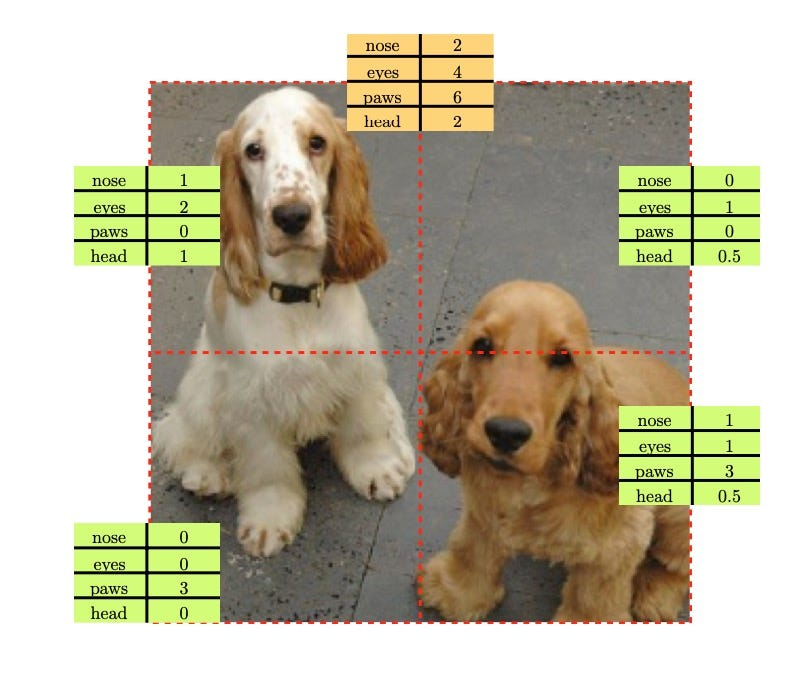# Representation Learning by Learning to Count

Source: Deep Learning on Medium# Representation Learning by Learning to Count

Transfer learning (aka Fine-tuning) is a core advantage of deep supervised learning. However, supervised learning requires labeled datasets which are expensive to acquire. Unsupervised/self-supervised learning is a cheaper alternative to supervised approaches. To avoid the costly annotation, an unsupervised learning approach leverages a pre-text task as a supervision signal. For example, Gidaris et al. rotate images and predict the rotation angle as a supervision signal. Similarly, Pathak et al. recover an image patch from the surrounding pixels. This paper  proposes a novel pre-text task for unsupervised learning.

The paper proposes a new simple, yet efficient, pre-text task, i.e., If we partition an image into non-overlapping regions, the number of visual primitives in each region should sum up to the number of primitives in the original image. The next figure shows that summing the count of visual primitives in an image’s tiles should match the count of visual primitives in the whole image. For example, The four tiles outline in red have {1,0,0,1} noses; accordingly, the whole image should have 2 noses. Concretely, The paper trains a neural network to count the number of visual primitives in an image.

Given an image x of size 228×228, the loss function of the counting pretext can be formulated as follow

where D(x) downsamples the image x by 2 into an image of size 114×114, T_i(x) indicates the tile #i, of size 114×114, from the original image x and N(.) is the network’s output representation.

This equation can be regarded as a contrastive loss, pulling the downsampled image representation D(x) closer to the representation from the four tiles T_i(x). One caveat, with the previous loss function, is that it has a trivial solution, i.e., N(.) equal zero. To avoid this solution, another term is added to include a “negative” image as follows

where [..]+ is max(0,..), M is scalar margin, y is a negative image randomly sampled. Basically, this triplet-loss style term pushes the learned representation of image x from that of image y. The next figure shows AlexNet architecture applied on two images (x,y).

where \phi symbol denotes the network’s output representation, i.e., it is N(.) in the previous two equations.

The counting network is trained using ImageNet datasets. The quality of the learned representation is quantitatively evaluated on three datasets: PASCAL, ImageNet, and Places. Using the PASCAL dataset, the pre-trained network, with the counting pretext, is fine-tuned on PASCAL VOC 2007 and VOC 2012. This is an established benchmark for object classification, detection and segmentation tasks as shown in the next table

The counting network is already trained on ImageNet dataset. Thus, for evaluation, a linear classifier on top of the frozen layers is trained on both ImageNet and Places. The next two tables present quantitative evaluation on both datasets

The paper provides further quantitative and qualitative evaluations that are omitted from this article.

1. There is a Tensorflow (TF) implementation on Github but not by the authors. This TF implementation employs VGG-16 instead of AlexNet! Thus, further modifications are required to use this code and compare results with related literature. I can only blame the paper’s authors, not Shao-Hua Sun, for that.
2. My experience with the code is not smooth. Expect to make further modifications.
3. The paper idea is simple and seems to work. I like simple ideas.
4. Many unsupervised learning approaches employ the same architecture, AlexNet, for quantitative evaluation. This is required for fair comparison but I wonder if these learning approaches remain valuable when applied to recent architectures like ResNet or DenseNet.
5. That being said, most papers probably adapt AlexNet, an old architecture, to make training and evaluation computationally cheaper. AlexNet is computationally feasible for a lot of scholars with limited GPU capability. Poor graduate students 🙂
6. There is one technical point that surprises me; the authors randomly pick either the bicubic, bilinear, Lanczos, or the area method to downsample the image. It is reported that this randomization of different downsampling methods significantly improves the detection performance by at least 2.2%!

Resources

 Representation Learning by Learning to Count

 Unsupervised Representation Learning by Predicting Image Rotations

 Context encoders: Feature learning by inpainting